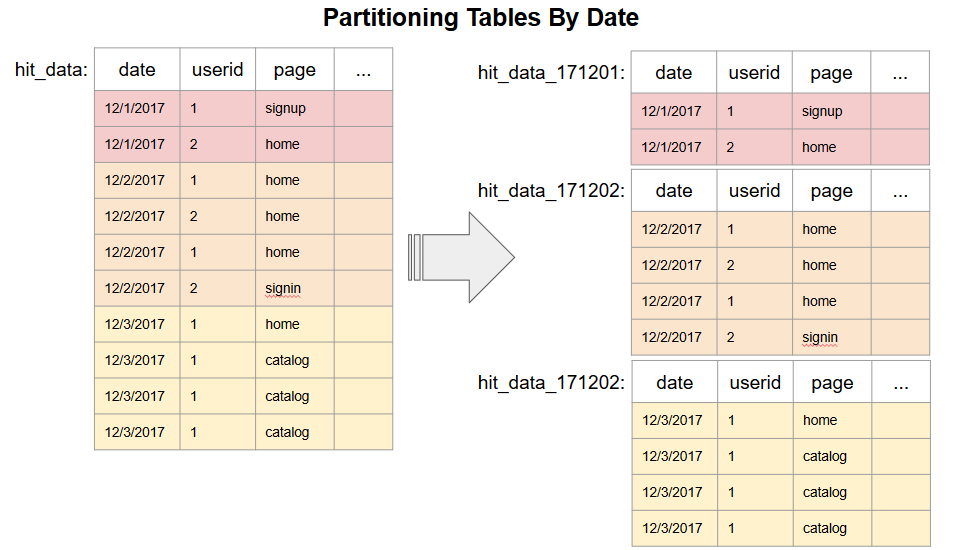# Cost-Effective BigQuery with R

by Roland Stevenson, Consultant

## Introduction

Companies using Google BigQuery for production analytics often run into the following problem: the company has a large user hit table that spans many years. Since queries are billed based on the fields accessed, and not on the date-ranges queried, queries on the table are billed for all available days and are increasingly wasteful.A solution is to partition the table by date, so that users can query a particular range of dates; saving costs and decreasing query duration. Partitioning an un-partitioned table can be expensive if done the brute-force way. This article explores one cost-effective partitioning method, and uses the condusco R Package to automate the query generation and partitioning steps.

## Migrating non-partitioned tables to partitioned tables in Google BigQuery

Let’s implement the accepted solution on StackOverflow for migrating from non-partitioned to partitioned tables in Google BigQuery.

The brute-force way to partition a non-partitioned table is to repeatedly query the table for anything matching a particular day and then save that data to a new table with the date suffix, ie. _20171201.

The problem is the cost for this method is the cost of querying the full table’s worth of data, multiplied by the number of days it needs to be partitioned into. For a 10 Terabyte table spanning three years, one SELECT * might cost $50 (BigQuery charges$5 per TB accessed). Hence, splitting the table into three years of daily partitions will cost $50*365*3 =$54,750!

The more cost-effective solution described on StackOverflow is to ARRAY_AGG the entire table into one record for each day. This requires one query over the table’s data to ARRAY_AGG each day you are interested in, and then multiple UNNEST queries using a single query on a single column.

This solution queries the full table’s worth of data twice, instead of the number of days. That’s a cost of $100, saving$54,650.

Here is an implementation of the solution using condusco to automate both the query generation and the partitioning:

library(whisker)
library(bigrquery)
library(condusco)

# Set GBQ project
project <- '<YOUR_GBQ_PROJECT_ID_HERE>'

# Configuration
config <- data.frame(
dataset_id = '<YOUR_GBQ_DATASET_ID_HERE>',
table_prefix = 'tmp_test_partition'
)

# Set the following options for GBQ authentication on a cloud instance
options("httr_oauth_cache" = "~/.httr-oauth")
options(httr_oob_default=TRUE)

# Run the below query to authenticate and write credentials to .httr-oauth file
query_exec("SELECT 'foo' as bar",project=project);

# The pipeline that creates the pivot table
migrating_to_partitioned_step_001_create_pivot <- function(params){

destination_table <- "{{{dataset_id}}}.{{{table_prefix}}}_partitions"

query <- "
SELECT
{{#date_list}}
ARRAY_CONCAT_AGG(CASE WHEN d = 'day{{{yyyymmdd}}}' THEN r END) AS day_{{{yyyymmdd}}},
{{/date_list}}
line
FROM (
SELECT d, r, ROW_NUMBER() OVER(PARTITION BY d) AS line
FROM (
SELECT
stn, CONCAT('day', year, mo, da) AS d, ARRAY_AGG(t) AS r
FROM bigquery-public-data.noaa_gsod.gsod2017 AS t
GROUP BY stn, d
)
)
GROUP BY line
"

query_exec(whisker.render(query,params),
project=project,
destination_table=whisker.render(destination_table, params),
write_disposition='WRITE_TRUNCATE',
use_legacy_sql = FALSE
);

}

# Run the pipeline that creates the pivot table

# Create a JSON string in the invocation query that looks like [{"yyyymmdd":"20171206"},{"yyyymmdd":"20171205"},...]
invocation_query <- "
SELECT
'{{{dataset_id}}}' as dataset_id,
'{{{table_prefix}}}' as table_prefix,
CONCAT(
'[',
STRING_AGG(
CONCAT('{\"yyyymmdd\":\"',FORMAT_DATE('%Y%m%d',partition_date),'\"}')
),
']'
) as date_list
FROM (
SELECT
DATE_ADD(DATE(CURRENT_DATETIME()), INTERVAL -n DAY) as partition_date
FROM (
SELECT [1,2,3] as n
),
UNNEST(n) AS n
)
"

run_pipeline_gbq(
migrating_to_partitioned_step_001_create_pivot,
whisker.render(invocation_query,config),
project,
use_legacy_sql = FALSE
)

# The pipeline that creates the individual partitions
migrating_to_partitioned_step_002_unnest <- function(params){

destination_table <- "{{{dataset_id}}}.{{{table_prefix}}}_{{{day_partition_date}}}"

query <- "
SELECT r.*
FROM {{{dataset_id}}}.{{{table_prefix}}}_partitions, UNNEST({{{day_partition_date}}}) as r
"

query_exec(whisker.render(query,params),
project=project,
destination_table=whisker.render(destination_table, params),
write_disposition='WRITE_TRUNCATE',
use_legacy_sql = FALSE
);

}

invocation_query <- "
SELECT
'{{{dataset_id}}}' as dataset_id,
'{{{table_prefix}}}' as table_prefix,
CONCAT('day_',FORMAT_DATE('%Y%m%d',partition_date)) as day_partition_date
FROM (
SELECT
DATE_ADD(DATE(CURRENT_DATETIME()), INTERVAL -n DAY) as partition_date
FROM (
SELECT [1,2,3] as n
),
UNNEST(n) AS n
)
"
run_pipeline_gbq(
migrating_to_partitioned_step_002_unnest,
whisker.render(invocation_query,config),
project,
use_legacy_sql = FALSE
)